Electron. J. Diff. Eqns.,Monograph 06, 2004 (142 pages)

### Palais-Smale approaches to semilinear elliptic equations in unbounded domains Hwai-chiuan Wang

Abstract:
Let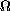be a domain in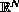,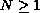, and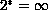if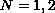,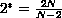if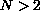,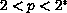.
Consider the semilinear elliptic problem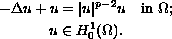Let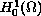be the Sobolev space in. The existence, the nonexistence, and the multiplicity of positive solutions are affected by the geometry and the topology of the domain. The existence, the nonexistence, and the multiplicity of positive solutions have been the focus of a great deal of research in recent years.
That the above equation in a bounded domain admits a positive solution is a classical result. Therefore the only interesting domains in which this equation admits a positive solution are proper unbounded domains. Such elliptic problems are difficult because of the lack of compactness in unbounded domains. Remarkable progress in the study of this kind of problem has been made by P. L. Lions. He developed the concentration-compactness principles for solving a large class of minimization problems with constraints in unbounded domains. The characterization of domains in which this equation admits a positive solution is an important open question. In this monograph, we present various analyses and use them to characterize several categories of domains in which this equation admits a positive solution or multiple solutions.

Submitted September 17, 2004. Published September 30, 2004.
Math Subject Classifications: 35J20, 35J25
Key Words: Palais-Smale condition; index; decomposition theorem; achieved domain; Esteban-Lions domain; symmtric Palais-Smale condition.

Show me the PDF file (1153K), TEX file, and other files for this article.Hwai-chiuan Wang Department of Mathematics National Tsing Hua University Hsinchu, Taiwan email: hwang@mail.math.nthu.edu.tw